Varsity Tutors always has a different Probability Theory Question of the Day ready at your disposal! If you’re just looking to get a quick review into your busy day, our Probability Theory Question of the Day is the perfect option. Answer enough of our Probability Theory Question of the Day problems and you’ll be ready to ace the next test. Check out what today’s Probability Theory Question of the Day is below.

### Question of the Day: Probability Theory

Let, and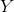be the lifespans (in hours) of two electronic devices, and their joint probability mass function is given below.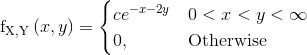Determine the value of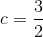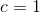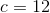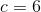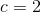### All Probability Theory Resources